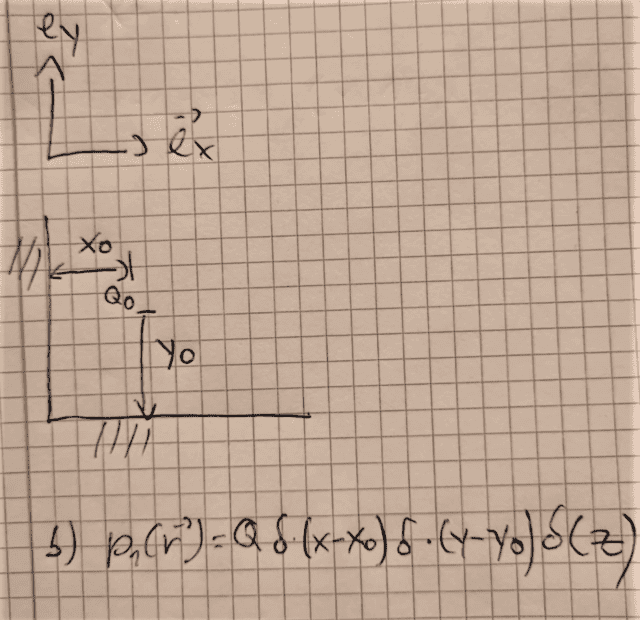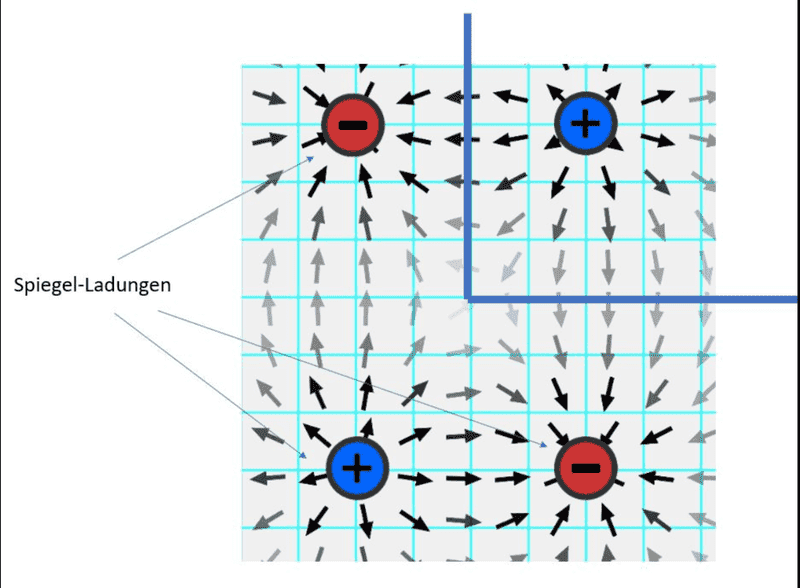# Sketch the Electric Field lines for a point charge near two conducting planes

Buzzer33
Homework Statement:
leaarning
Relevant Equations:
no
Guys I have Problems with this task The arrangement consists of a point charge Q at a distance (x0, y0,0) from the origin and two perfectly conductive surfaces in the (x, z) and (y, z) plane

a) Mathematical description of the space charge density p of the original and mirror charge using the delta function. Consider the perfectly conductive surfaces as infinitely extended in the respective direction and carry out reflections. b) Calculate the potential

c) Draw lines E field

Has somebody ideas for a)and b) because I am learning currently

Here the sketch to the task

https://ibb.co/zbSyb3d

Mentor
Homework Statement:: leaarning
Relevant Equations:: no

Guys I have Problems with this task The arrangement consists of a point charge Q at a distance (x0, y0,0) from the origin and two perfectly conductive surfaces in the (x, z) and (y, z) plane

a) Mathematical description of the space charge density p of the original and mirror charge using the delta function. Consider the perfectly conductive surfaces as infinitely extended in the respective direction and carry out reflections. b) Calculate the potential

c) Draw lines E field

Has somebody ideas for a)and b) because I am learning currently

Here the sketch to the task

https://ibb.co/zbSyb3d
Welcome to PF.

The picture of your sketch is pretty fuzzy, but it looks like it is a single point charge that is located a distance awat from two orthogonal conducting planes? If you have a better picture or can post an image of the actual problem statement, that would help.

Also, you need to show your work before we can offer tutorial help.

And what do you know about the E-field lines for a point charge above a single conducting plane? What does it mean to use a "mirror charge" image, and how does that simplify the problem?

Buzzer33
I made the sketch a little better .I haven't got a better on .

I did a little work in b) which is in the pic but don't know what to do furtherhttps://ibb.co/0CqtSqY

Homework Helper
Gold Member
2022 Award
I made the sketch a little better .I haven't got a better on .

I did a little work in b) which is in the pic but don't know what to do furtherView attachment 289170

https://ibb.co/0CqtSqY
I can't read the LHS of the equation, but the RHS looks right for the space charge density of the point charge.
Please post algebra as typed in, not in images (per forum rules). Use LaTeX if you can. There's a guide at the link at bottom left.

What about the mirror charges? Where are they, and what are their signs?
Hint: it's very like looking at your own reflections in two mirrors at right angles.

•berkeman
Buzzer33
Do you know how to draw line E field ?

https://ibb.co/6DxjK2WLast edited by a moderator: